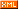# Idiot-maker

 :: 首页 :: 博问 :: 闪存 :: 新随笔 :: 联系 :: 订阅:: 管理 ::https://leetcode.com/problems/rotate-array/

Given an array, rotate the array to the right by k steps, where k is non-negative.

Example 1:

Input: [1,2,3,4,5,6,7] and k = 3
Output: [5,6,7,1,2,3,4]
Explanation:
rotate 1 steps to the right: [7,1,2,3,4,5,6]
rotate 2 steps to the right: [6,7,1,2,3,4,5]
rotate 3 steps to the right: [5,6,7,1,2,3,4]


Example 2:

Input: [-1,-100,3,99] and k = 2
Output: [3,99,-1,-100]
Explanation:
rotate 1 steps to the right: [99,-1,-100,3]
rotate 2 steps to the right: [3,99,-1,-100]


Note:

• Try to come up as many solutions as you can, there are at least 3 different ways to solve this problem.
• Could you do it in-place with O(1) extra space?

O(nk)的解法

class Solution {
public void rotate(int[] nums, int k) {
k = k % nums.length;
while (k > 0) {
int temp = nums[nums.length - 1];
for (int i = nums.length - 1; i > 0; i--) {
nums[i] = nums[i - 1];
}
nums = temp;
k--;
}
}
}

public void rotate(int[] nums, int k) {
k %= nums.length;
reverse(nums, 0, nums.length - 1);
reverse(nums, 0, k - 1);
reverse(nums, k, nums.length - 1);
}

public void reverse(int[] nums, int start, int end) {
while (start < end) {
int temp = nums[start];
nums[start] = nums[end];
nums[end] = temp;
start++;
end--;
}
}

posted on 2018-12-25 00:36  NickyYe  阅读(96)  评论(0编辑  收藏  举报